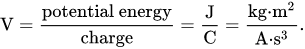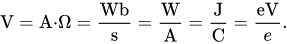# Gigavolts (GV) to volts (V) free online conversion

Kilovolts (kV) to volts (V) conversion - calculator and how to convert.

## Gigavolts to volts conversion calculator

Enter the voltage in gigavolts and press the Convert button:

GV
V

Volts to GV free online conversion »

## How to convert gigavolts to volts

1 GV = 109 V = 1000000000 V

or

1 V = 10-9 GV = 0.000000001 GV

#### Gigavolts to volts formula

The voltage V in gigavolts (GV) is equal to the voltage V in volts (V) times 1000000000:

V(V) = V(GV) × 1000000000

#### Example

Convert 3 kilovolts to volts:

V(V) = 3GV × 109 = 3×109 V

## Gigavolts to volts conversion table

Gigavolts (GV) Volts (V)
0 GV 0 V
0,000001 GV 103 V
0,00001 GV 104 V
0,0001 GV 105 V
0,001 GV 106 V
0,01 GV 107 V
0,1 GV 108 V
1 GV 109 V

## Volt definition

One volt is defined as the electric potential between two points of a conducting wire when an electric current of one ampere dissipates one watt of power between those points. Equivalently, it is the potential difference between two points that will impart one joule of energy per coulomb of charge that passes through it. It can be expressed in terms of SI base units (m, kg, s, and A) as:It can also be expressed as amperes times ohms (current times resistance, Ohm's law), webers per second (magnetic flux per time), watts per ampere (power per unit current, definition of electric power), or joules per coulomb (energy per unit charge), which is also equivalent to electronvolts per elementary charge:Volts to GV free online conversion »

Currently, we have around 5612 calculators, conversion tables and usefull online tools and software features for students, teaching and teachers, designers and simply for everyone.